Trigonometry : Identities of Inverse Operations

Example Questions

Example Question #71 : Trigonometric Identities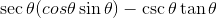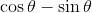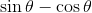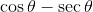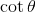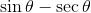Explanation:

The easiest first step is to simplify our inverse identities: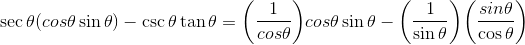Cross cancelling, we end up with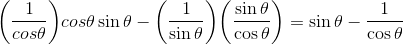Finally, eliminate the fraction: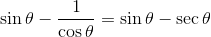Thus,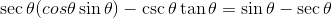Example Question #72 : Trigonometry

Simplify each expression below. Your answer should have (at most) one trigonometric function and no fractions.

1.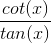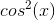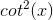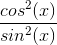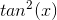Explanation:

Using the quotient identities for trig functions, you can rewrite,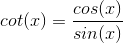and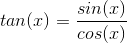Then the fraction becomes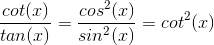Example Question #2 : Identities Of Inverse Operations

Simplify each expression below. Your answer should have (at most) one trigonometric function and no fractions.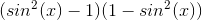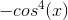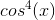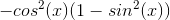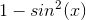Explanation:

Use the Pythagorean Identities: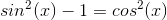and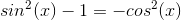Thus the expression becomes,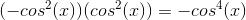.

Example Question #74 : Trigonometry

Simplify each expression below. Your answer should have (at most) one trigonometric function and no fractions.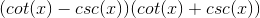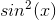Explanation:

Use the distributive property (FOIL method) to simplify the expression.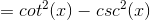Using Pythagorean Identities: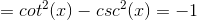.

Example Question #75 : Trigonometry

Simplify each expression below. Your answer should have (at most) one trigonometric function and no fractions.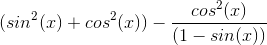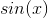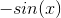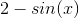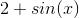Explanation:

First, simplify the first term in the expression to 1 because of the Pythagorean Identity.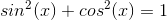Then, simplify the second term to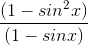.

This reduces to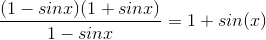.

The expression is now,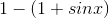.

Distribute the negative and get,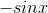.

Example Question #76 : Trigonometry

Solve each question over the interval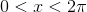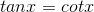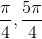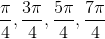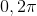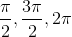Explanation:

Divide both sides by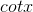to get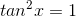.

Take the square root of both sides to get that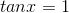and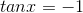.

The angles for which this is true (this is taking the arctan) are every angle when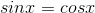and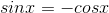.

These angles are all the multiples of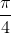Example Question #77 : Trigonometry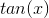can be stated as all of the following except...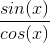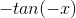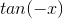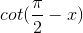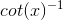Explanation:

Let's look at these individually:is true by definition, as is.is also true because of a co-function identity.

This leaves two - and we can tell which of these does not work using the fact that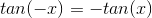, which means thatis our answer.